#### Friday - February 5th### Friday, February 5th

All times are in Central European Time (CET). Underlined authors are presenting the talks.
Click on the talk title to access the recording.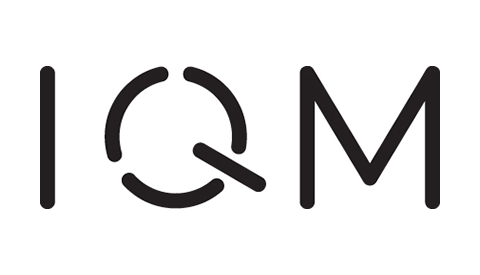Sessions on Friday are sponsored by IQM.

### Monday | Tuesday | Wednesday | Thursday

##### 07:30 - 08:30 | Mentoring Session #3

Prior registration is required and only available for QIP 2021 participants. Specific times and dates are sent out via email.

### Session 1A

Main stage (A)Authors: Yuval Sanders, Dominic Berry, Pedro Costa, Louis Tessler, Nathan Wiebe, Craig Gidney, Hartmut Neven and Ryan Babbush

Abstract

Affiliations: Macquarie University | Macquarie University | Macquarie University | Macquarie University | University of Washington | Google | Google | Google

Extended abstract

Abstract
We compile explicit circuits and evaluate the computational cost for heuristic-based quantum algorithms for combinatorial optimization. We consider several variants of quantum-accelerated simulated annealing as well as adiabatic algorithms, quantum-enhanced population transfer, the quantum approximate optimization algorithm, and other approaches. We provide novel methods for executing the bottleneck subroutines for these heuristics, and our methods can easily be applied to other algorithms where numerical performance matters. We estimate how quickly the subroutines could be executed on a modestly sized superconducting-qubit-based quantum computer with surface code error correction. We conclude that quadratic speedups for heuristic-based quantum optimization algorithms are insufficient for early quantum computers to beat present day classical computers.Authors: Daniel Stilck França and Raul Garcia-Patron

Abstract

Affiliations: University of Copenhagen | University of Edinburgh

Abstract
Recent technological developments have focused the interest of the quantum computing community on investigating how near-term devices could outperform classical computers for practical applications. A central question that remains open is whether their noise can be overcome or it fundamentally restricts any potential quantum advantage. We present a transparent way of comparing classical algorithms to quantum ones running on near-term quantum devices for a large family of problems that include optimization problems and approximations to the ground state energy of Hamiltonians. Our approach is based on the combination of entropic inequalities that determine how fast the quantum computation state converges to the fixed point of the noise model, together with established classical methods of Gibbs state sampling. The approach is extremely versatile and allows for its application to a large variety of problems, noise models and quantum computing architectures. We use our results to provide estimates for a variety of problems and architectures that have been the focus of recent experiments, such as quantum annealers, variational quantum eigensolvers, and quantum approximate optimization. The bounds we obtain indicate that substantial quantum advantages are unlikely for classical optimization unless the current noise rates are decreased by orders of magnitude or the topology of the problem matches that of the device. This is the case even if the number of qubits increases substantially. We reach similar but less stringent conclusions for quantum Hamiltonian problems.Authors: Kun Fang and Zi-Wen Liu // Bartosz Regula and Ryuji Takagi

Abstract

Affiliations: University of Waterloo | Perimeter Institute for Theoretical Physics // Nanyang Technological University | Nanyang Technological University

Abstract
The manipulation of quantum resources such as entanglement and coherence lies at the heart of quantum science and technology, empowering potential advantages over classical methods. In practice, a particularly important kind of manipulation is to purify the quantum resources, since they are inevitably contaminated by noises and thus often lost their power or become unreliable for direct usage. In these two works, we establish a theory of the universal limitations on the accuracy and efficiency of resource purification tasks which apply to any well-behaved resource theory, for both state (static) and channel (dynamical) resources. The general results bring new insights and imply various forms of fundamental limits to a broad range of problems of great theoretical and practical importance, including magic state distillation and fault tolerant quantum computing, quantum error correction, quantum Shannon theory, and quantum circuit synthesis. // We establish universal limitations on the manipulation of quantum channel resources under the most general transformation protocols. Focusing in particular on the class of distillation tasks -- which can be understood either as the purification of noisy channels into unitary ones, or the extraction of state-based resources from channels -- we develop fundamental restrictions on the error necessarily incurred in such transformations. Our results are applicable to the study of general quantum resources under any physical manipulation scheme, which includes general adaptive protocols with or without a definite causal order. We introduce comprehensive lower bounds for the overhead of any distillation protocol in terms of required channel uses, imposing strong limitations on the practical efficiency and cost of channel resource manipulation. In the asymptotic setting, our results yield broadly applicable strong converse bounds for the rates of distillation. As a special case, our methods apply to the manipulation of quantum states, in which case they significantly improve on and extend previous approaches. We demonstrate our results through explicit applications to quantum communication, where we recover in particular a number of strong converse bounds for the quantum capacity of channels assisted by different classes of operations, as well as to fault-tolerant quantum computation, where we obtain improved bounds for the overhead cost of magic state distillation and gate synthesis.

10:00 - 10:30
Round tables with Yuval Sanders, Daniel Stilck França, Zi-Wen Liu, and Bartosz Regula
At the end of the session, you can meet with the speakers directly. Round tables take place in parallel, each speaker having their own meeting.

### Session 1B

Stage B08:30 - 09:00
Almost Public Quantum Coins

Authors: Amit Behera and Or Sattath

Abstract

Affiliations: Ben-Gurion University of the Negev, Israel | Ben-Gurion University of the Negev, Israel

Extended abstract

Abstract
In a quantum money scheme, a bank can issue money that users cannot counterfeit. Similar to bills of paper money, most quantum money schemes assign a unique serial number to each money state, thus potentially compromising the privacy of the users of quantum money. However in a quantum coins scheme, just like the traditional currency coin scheme, all the money states are exact copies of each other, providing a better level of privacy for the users. A quantum money scheme can be private, i.e., only the bank can verify the money states, or public, meaning anyone can verify. In this work, we propose a way to lift any private quantum coin scheme -- which is known to exist based on the existence of one-way functions by Ji, Liu, and Song (CRYPTO'18) -- to a scheme that closely resembles a public quantum coin scheme. Verification of a new coin is done by comparing it to the coins the user already possesses, by using a projector on to the symmetric subspace. No public coin scheme was known prior to this work. It is also the first construction that is close to a public quantum money scheme and is provably secure based on standard assumptions. The lifting technique when instantiated with the private quantum coins scheme by Mosca and Stebila (2010) gives rise to the first construction that is close to an inefficient unconditionally secure public quantum money scheme.09:00 - 09:30
Distributed Quantum Proofs for Replicated Data

Authors: Pierre Fraigniaud, Francois Le Gall, Harumichi Nishimura and Ami Paz

Abstract

Affiliations: CNRS and Universite de Paris | Nagoya University | Nagoya University | Universitat Wien

Extended abstract

Abstract
This paper tackles the issue of checking that all copies of a large data set replicated at several nodes of a network are identical. The fact that the replicas may be located at distant nodes prevents the system from verifying their equality locally, i.e., by having each node consult only nodes in its vicinity. On the other hand, it remains possible to assign certificates to the nodes, so that verifying the consistency of the replicas can be achieved locally. However, we show that, as the replicated data is large, classical certification mechanisms, including distributed Merlin-Arthur protocols, cannot guarantee good completeness and soundness simultaneously, unless they use very large certificates. The main result of this paper is a distributed quantum Merlin-Arthur protocol enabling the nodes to collectively check the consistency of the replicas, based on small certificates, and in a single round of message exchange between neighbors, with short messages. In particular, the certificate-size is logarithmic in the size of the data set, which gives an exponential advantage over classical certification mechanisms. We propose yet another usage of a fundamental quantum primitive, called the SWAP test, in order to show our main result.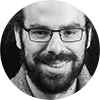Authors: Alex Bredariol Grilo, Kathrin Hövelmanns, Andreas Hülsing and Christian Majenz

Abstract

Affiliations: LIP6, CNRS/Sorbonne Université | Ruhr-Universität Bochum | Eindhoven University of Technology | CWI and QuSoft

Extended abstract

Abstract
The random oracle model (ROM) enjoys widespread popularity, mostly because it tends to allow for tight and conceptually simple proofs where provable security in the standard model is elusive or costly. While being the adequate replacement of the ROM in the post-quantum security setting, the quantum-accessible random oracle model (QROM) has thus far failed to provide these advantages in many settings. In this work, we focus on adaptive reprogrammability, a feature of the ROM enabling tight and simple proofs in many settings. We show that the straightforward quantum-accessible generalization of adaptive reprogramming is feasible by proving a bound on the adversarial advantage in distinguishing whether a random oracle has been reprogrammed or not. We show that our bound is tight by providing a matching attack. We go on to demonstrate that our technique recovers the mentioned advantages of the ROM in three QROM applications: 1) We give a tighter proof of security of the message compression routine as used by XMSS. 2) We show that the standard ROM proof of chosen-message security for Fiat-Shamir signatures can be lifted to the QROM, straightforwardly, achieving a tighter reduction than previously known. 3) We give the first QROM proof of security against fault injection and nonce attacks for the hedged Fiat-Shamir transform.

10:00 - 10:30
Round tables with Amit Behera, Harumichi Nishimura, and Christian Majenz
At the end of the session, you can meet with the speakers directly. Round tables take place in parallel, each speaker having their own meeting.

### Session 1C

Stage CAuthors: Hsin-Yuan Huang, Richard Kueng, Michael Broughton, Masoud Mohseni, Ryan Babbush, Sergio Boixo, Hartmut Neven, Jarrod McClean and John Preskill

Abstract

Extended abstract

Abstract
Machine learning (ML) provides the potential to solve challenging quantum many-body problems in physics and chemistry. Yet, this prospect has not been fully justified. In this work, we establish rigorous results to understand the power of classical ML and the potential for quantum advantage in an important example application: predicting outcomes of quantum mechanical processes. We prove that for achieving a small average prediction error, one can always design a classical ML model whose sample complexity is comparable to the best quantum ML model (up to a small polynomial factor). Regarding computational complexity, we show that the class of problems that can be solved by efficient classical ML models with access to sampled data is strictly larger than BPP. Hence, classical ML models may be able to solve some challenging quantum problems after training from data obtained in physical experiments. As a concrete example, we prove that a simple, classical ML model can efficiently learn to predict ground state representations that approximate expectation values of local observables up to a small, constant error. This holds for any smooth family of gapped local Hamiltonians in a finite spatial dimension.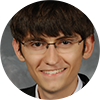09:00 - 09:30
Symmetries, graph properties, and quantum speedups

Authors: Shalev Ben-David, Andrew M. Childs, András Gilyén, William Kretschmer, Supartha Podder and Daochen Wang

Abstract

Affiliations: University of Waterloo | University of Maryland | California Institute of Technology | University of Texas at Austin | University of Ottawa | University of Maryland

Extended abstract

Abstract
Aaronson and Ambainis (2009) and Chailloux (2018) showed that fully symmetric (partial) functions do not admit exponential quantum query speedups. This raises a natural question: how symmetric must a function be before it cannot exhibit a large quantum speedup? In this work, we prove that hypergraph symmetries in the adjacency matrix model allow at most a polynomial separation between randomized and quantum query complexities. We also show that, remarkably, permutation groups constructed out of these symmetries are essentially the only permutation groups that prevent super-polynomial quantum speedups. We prove this by fully characterizing the primitive permutation groups that allow super-polynomial quantum speedups. In contrast, in the adjacency list model for bounded-degree graphswhere graph symmetry is manifested differentlywe exhibit a property testing problem that shows an exponential quantum speedup. These results resolve open questions posed by Ambainis, Childs, and Liu (2010) and Montanaro and de Wolf (2013).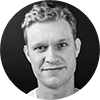Authors: Simon Apers, Troy Lee and Ronald de Wolf

Abstract

Affiliations: ULB - CWI | University of Technology, Sydney | QuSoft, CWI and University of Amsterdam (Amsterdam)

Extended abstract

Abstract
Graph sparsification underlies a large number of algorithms for graph cut problems and solving Laplacian systems. In its strongest form, "spectral sparsification" reduces the number of edges to near-linear in the number of nodes, while approximately preserving the cut and spectral structure of the graph. We give a quantum algorithm that outputs a classical description of an $\varepsilon$-spectral sparsifier of a weighted graph with $n$ vertices and $m$ edges. It has time complexity $\widetilde O(\sqrt{mn}/\varepsilon)$ in the adjacency array model and $\widetilde O(n^{3/2}/\varepsilon)$ in the adjacency matrix model. These bounds are tight up to polylogarithmic factors, and improve on the optimal classical complexities $\Omega(m)$ and $\Omega(n^2)$, respectively. Using classical algorithms on the obtained sparsifier yields immediate quantum speedups for approximately solving Laplacian systems and for approximating a range of graph cut problems in essentially the same complexity. As a significantly more involved application we show how to speed up the quantum query complexity for computing exactly the edge connectivity of simple graphs. We show upper bounds on the query complexity of $\widetilde O(\sqrt{mn})$ and $\widetilde O(n^{3/2})$ in the adjacency matrix and adjacency array models, respectively. The upper bound for the adjacency matrix model is tight up to logarithmic factors, while for the adjacency array model the best quantum query lower bound we know is $\Omega(n)$.

10:00 - 10:30
Round tables with Hsin-Yuan Huang, William Kretschmer, and Simon Apers
At the end of the session, you can meet with the speakers directly. Round tables take place in parallel, each speaker having their own meeting.

##### 10:45 - 11:00 | Digital group photo

We would like to commemorate the QIP2021 with the entire community and invite you to join us for a group photo op. This will happen in a Zoom meeting; the link will be shared in the #chit-chat_at_qip Slack channel a few minutes before the event.

### Session 2A

Main stage (A)11:00 - 11:30
Multi-port teleportation schemes

Authors: Piotr Kopszak, Marek Mozrzymas, Michal Studzinski and Michal Horodecki

Abstract

Affiliations: University of Wroclaw | University of Wroclaw | University of Gdansk | International Centre for Theory of Quantum Technologies, University of Gdansk

Abstract
We introduce and discuss a novel multi-port based teleportation schemes performing transmission of a number of unknown quantum states or one composite system in one go. We fully characterize the probabilistic and deterministic case by presenting expressions for the average probability of success and entanglement fidelity in both non-optimal and optimal variant. We also deliver explicit forms of the measurements and the resource state exploited by parties to perform the process. To obtain our results, i.e. explicit expressions for the performance of the new schemes, we deliver novel mathematical tools concerning representation theory of the algebra of partially transposed permutation operators, where the transposition acts on more than one subsystem. Additionally, the optimal values of the entanglement fidelity and probability success emerge from formulated and solved primal and dual semidefinite problems, which due to existing symmetries and delivered mathematical tools could be solved analytically. Next, we have applied the obtained formulas for the performance of multi-port based teleportation schemes to get a qualitative improvement of asymptotic "teleportation capacities" of multi-port based teleportation schemes over the pre-existing port-based teleportation schemes.11:30 - 12:00
Optimal universal programming of unitary gates

Authors: Yuxiang Yang, Renato Renner and Giulio Chiribella

Abstract

Affiliations: Institute for Theoretical Physics, ETH Zurich | Institute for Theoretical Physics, ETH Zurich | The University of Hong Kong

Extended abstract

Abstract
A universal quantum processor is a device that takes as input a (quantum) program, containing an encoding of an arbitrary unitary gate, and a (quantum) data register, on which the encoded gate is applied. While no perfect universal quantum processor can exist, approximate processors have been proposed in the past two decades. A fundamental open question is how the size of the smallest quantum program scales with the approximation error. Here we answer the question, by proving a bound on the size of the program and designing a concrete protocol that attains the bound in the asymptotic limit. Our result is based on a connection between optimal programming and the Heisenberg limit of quantum metrology, and establishes an asymptotic equivalence between the tasks of programming, learning, and estimating unitary gates.Authors: Tanmay Singal, Filip Maciejewski and Michał Oszmaniec

Abstract

Affiliations: Center for Theoretical Physics, Polish Academy of Sciences | Center for Theoretical Physics, Polish Academy of Sciences | Center for Theoretical Physics, Polish Academy of Sciences

Extended abstract

Abstract
It is imperative to minimize resources needed to implement quantum operations on existing near-term quantum devices. With this in mind, we propose a scheme to implement an arbitrary general quantum measurement, also known as Positive Operator Valued Measures (POVM) in dimension d using only classical resources and a single ancillary qubit. The proposed method is based on probabilistic implementation of d outcome measurements which is followed by postselection on some of the received outcomes. This is an extension of an earlier work which required dichotomic measurements, no additional ancillary qubits, and whose success probability scaled like 1/d. The success probability of our scheme depends on the operator norms of the coarse grained POVM effects. Significantly, we show that for typical Haar random rank-one POVMs with at most d 2 outcomes, the success probability of our simulation scheme does not go to zero with the dimension of the system. We conjecture that this is true for all POVMs in dimension d. This is supported by numerical computations showing constant success probability for SIC-POVMs and (non symmetric) IC-POVMs in dimensions up to 323. Additionally, for the gate noise model used in the recent demonstration of quantum computational advantage by Google, we prove that for typical Haar random POVMs noise compounding in circuits required by our scheme is substantially lower than in the scheme that directly uses Naimarks dilation theorem.

12:30 - 13:00

Round tables with Piotr Kopszak, Michal Studzinski, Yuxiang Yang, Tanmay Singal, and Filip Maciejewski
At the end of the session, you can meet with the speakers directly. Round tables take place in parallel, each speaker having their own meeting.

### Session 2B

Stage B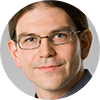Authors: Arne Heimendahl, Markus Heinrich and David Gross

Abstract

Affiliations: University of Cologne | Universität Köln | Universität Köln

Extended abstract

Abstract
Stabiliser operations occupy a prominent role in the theory of fault-tolerant quantum computing. They are defined operationally: by the use of Clifford gates, Pauli measurements and classical control. Within the stabiliser formalism, these operations can be efficiently simulated on a classical computer, a result which is known as the Gottesman-Knill theorem. However, an additional supply of magic states is enough to promote them to a universal, fault-tolerant model for quantum computing. To quantify the needed resources in terms of magic states, a resource theory of magic has been developed during the last years. Stabiliser operations (SO) are considered free within this theory, however they are not the most general class of free operations. From an axiomatic point of view, these are the completely stabiliser-preserving (CSP) channels, defined as those that preserve the convex hull of stabiliser states. It has been an open problem to decide whether these two definitions lead to the same class of operations. In this work, we answer this question in the negative, by constructing an explicit counter-example. This indicates that recently proposed stabiliser-based simulation techniques of CSP maps might be strictly more powerful than Gottesman-Knill-like methods. The result is analogous to a well-known fact in entanglement theory, namely that there is a gap between the class of local operations and classical communication (LOCC) and the class of separable channels. Along the way, we develop a number of auxiliary techniques which allow us to better characterise the set of CSP channels.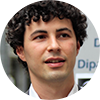11:30 - 12:00
Entangleability of cones

Authors: Guillaume Aubrun, Ludovico Lami, Carlos Palazuelos and Martin Plávala

Abstract

Affiliations: Université Lyon 1 | Ulm University | Departamento de Análisis Matemático y Matemática Aplicada, Universidad Complutense de Madrid | University of Siegen

Extended abstract

Abstract
We prove that two non-classical general probabilistic theories must give rise to entanglement, either at the level of states or at the level of measurements, when combined. This reveals a deep connection between a local phenomenon (non-classicality, or the existence of superpositions) and a global one (entanglement), and raises the latter to a generically non-classical rather than merely quantum phenomenon, in a precise mathematical sense. Instrumental in our proof is the solution of a long-standing conjecture by Barker.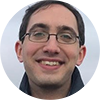Abstract

Affiliations: University of Oxford | University of Nottingham | University of Nottingham

Extended abstract

Abstract
The classical Gibbs paradox concerns the entropy change upon mixing two gases. Whether an observer assigns an entropy increase to the process depends on their ability to distinguish the gases. A resolution is that an ignorant' observer, who cannot distinguish the gases, has no way of extracting work by mixing them. Moving the thought experiment into the quantum realm, we reveal new and surprising behaviour: the ignorant observer can extract work from mixing different gases, even if the gases cannot be directly distinguished. Moreover, in the macroscopic limit, the quantum case diverges from the classical ideal gas: as much work can be extracted as if the gases were fully distinguishable. We show that the ignorant observer assigns more microstates to the system than found by naive counting in semiclassical statistical mechanics. This demonstrates the importance of accounting for the level of knowledge of an observer, and its implications for genuinely quantum modifications to thermodynamics.

12:30 - 13:00
Round table with Markus Heinrich, Ludovico Lami, and Benjamin Yadin
At the end of the session, you can meet with the speakers directly. Round tables take place in parallel, each speaker having their own meeting.

### Session 2C

Stage CAuthors: Troy Lee, Miklos Santha and Shengyu Zhang // Ashley Montanaro and Changpeng Shao

Abstract

Affiliations: PhaseCraft Ltd and University of Bristol | School of Mathematics, University of Bristol // University of Technology, Sydney | CNRS, IRIF, Université de Paris and CQT Singapore | Tencent Quantum Laboratory

Abstract
Let $G$ be an $n$-vertex graph with $m$ edges. When asked a subset $S$ of vertices, a cut query on $G$ returns the number of edges of $G$ that have exactly one endpoint in $S$. We show that there is a bounded-error quantum algorithm that determines all connected components of $G$ after making $O(\log(n)^6)$ many cut queries. In contrast, it follows from results in communication complexity that any randomized algorithm even just to decide whether the graph is connected or not must make at least $\Omega(n/\log(n))$ many cut queries. We further show that with $O(\log(n)^8)$ many cut queries a quantum algorithm can with high probability output a spanning forest for $G$. En route to proving these results, we design quantum algorithms for learning a graph using cut queries. We show that a quantum algorithm can learn a graph with maximum degree $d$ after $O(d \log(n)^2)$ many cut queries, and can learn a general graph with $O(\sqrt{m} \log(n)^{3/2})$ many cut queries. These two upper bounds are tight up to the poly-logarithmic factors, and compare to $\Omega(dn)$ and $\Omega(m/\log(n))$ lower bounds on the number of cut queries needed by a randomized algorithm for the same problems, respectively. The key ingredients in our results are the Bernstein-Vazirani algorithm, approximate counting with OR queries'', and learning sparse vectors from inner products as in compressed sensing. // We study the problem of learning an unknown graph provided via an oracle using a quantum algorithm. We consider three query models. In the first model (OR queries''), the oracle returns whether a given subset of the vertices contains any edges. In the second (parity queries''), the oracle returns the parity of the number of edges in a subset. In the third model, we are given copies of the graph state corresponding to the graph. We give quantum algorithms that achieve speedups over the best possible classical algorithms in the OR and parity query models, for some families of graphs, and give quantum algorithms in the graph state model whose complexity is similar to the parity query model. For some parameter regimes, the speedups can be exponential in the parity query model. On the other hand, without any promise on the graph, no speedup is possible in the OR query model. A main technique we use is the quantum algorithm for solving the combinatorial group testing problem, for which a query-efficient quantum algorithm was given by Belovs. Here we additionally give a time-efficient quantum algorithm for this problem, based on the algorithm of Ambainis et al.\ for a gapped" version of the group testing problem. We also give simple time-efficient quantum algorithms based on Fourier sampling and amplitude amplification for learning the exact-half and majority functions, which almost match the optimal complexity of Belovs' algorithms.Authors: Ankit Garg, Robin Kothari, Praneeth Netrapalli and Suhail Sherif

Abstract

Affiliations: Microsoft | Microsoft | Microsoft | Tata Institute of Fundamental Research

Extended abstract

Abstract
We study the first-order convex optimization problem, where we have black-box access to a (not necessarily smooth) function f:R^n->R and its (sub)gradient. Our goal is to find an eps-approximate minimum of f starting from a point that is distance at most R from the true minimum. If f is G-Lipschitz, then the classic gradient descent algorithm solves this problem with O((GR/eps)^2) queries. Importantly, the number of queries is independent of the dimension n and gradient descent is optimal in this regard: No deterministic or randomized algorithm can achieve better complexity that is still independent of the dimension n. In this paper we reprove the matching randomized lower bound using a simpler argument than previous lower bounds. We then show that although the function family used in the lower bound is hard for randomized algorithms, it can be solved quadratically faster using quantum queries. We then show an improved lower bound against quantum algorithms using a different set of instances and establish our main result that in general even quantum algorithms need Omega((GR/eps)^2) queries to solve the problem. Hence there is no quantum speedup over gradient descent for black-box first-order convex optimization without further assumptions on the function family. In our second result, we consider the case of smooth functions. Here the optimal classical algorithm is not gradient descent, but accelerated gradient descent, which is also known to be optimal among all classical (randomized) algorithms. We show a matching quantum lower bound, showing that there is no quantum speedup over accelerated gradient descent either.Authors: Jintai Ding, Vlad Gheorghiu, András Gilyén, Sean Hallgren and Jianqiang Li

Abstract

Affiliations: University of Cincinnati, OH, USA | softwareQ Inc. / Institute for Quantum Computing / Dept.\ of Combinatorics \& Optimization, University of Waterloo | California Institute of Technology | Department of Computer Science and Engineering, Pennsylvania

Abstract
Recently Chen and Gao~\cite{ChenGao2017} proposed a new quantum algorithm for Boolean polynomial system solving, motivated by the cryptanalysis of some post-quantum cryptosystems. The key idea of their approach is to apply a Quantum Linear System (QLS) algorithm to a Macaulay linear system over $\CC$, which is derived from the Boolean polynomial system. The efficiency of their algorithm depends on the condition number of the Macaulay matrix. In this paper, we give a strong lower bound on the condition number as a function of the Hamming weight of the solution. We describe a Grover-based exhaustive search algorithm that always outperforms their algorithm. Then, we improve upon Chen and Gao's algorithm by introducing the Boolean Macaulay linear system over $\CC$ by reducing the original Macaulay linear system. This improved algorithm could potentially significantly outperform the brute-force algorithm, when the Hamming weight of the solution is logarithmic in the number of variables. Furthermore, we provide a simple and more elementary proof of correctness for our improved algorithm using a reduction employing the Valiant-Vazirani affine hashing method, and also extend the result to polynomial systems over $\FF_q$ improving on subsequent work by Chen, Gao and Yuan \cite{ChenGao2018}. We also suggest a new approach for extracting the solution of the Boolean polynomial system via a generalization of the quantum coupon collector problem \cite{arunachalam2020quantum}.

12:30 - 13:00
Round table with Troy Lee, Changpeng Shao, Suhail Sherif, and Jianqiang Li
At the end of the session, you can meet with the speakers directly. Round tables take place in parallel, each speaker having their own meeting.

##### 14:00 on QIP TV | Lab tour "Quantum Technologies Lab" with Timo Sommer and David Hoch

Join the QIP TV stage for an insight of the work done by Menno Poot's group at Department of Physics - TUM in Garching near Munich.

### Session 3

Main stage (A)##### 15:00 - 16:00 | Challenges and Directions of Quantum Information Technology

Authors: Jay Gambetta

Abstract

Affiliation: IBM Fellow and VP of Quantum Computing, IBM Quantum

Abstract
In the past decade, the quantum computing community has expanded from a small,research-focused group of physicists, engineers and mathematicians to a large andinterdisciplinary field that includes experts from all domains including industry,government, and academia. As a result, we have seen accelerated progress towardunderstanding the scope of quantum computing, pushing its hardware technology,developing applications, and advancing error correction protocols. In this talk, I wouldlike to share my five-year vision for advancing quantum information technology, includinghow we will push the limits and what the key challenges to realizing frictionless quantumcomputing are—that is, quantum computing integrated seamlessly with high performancecomputing resources.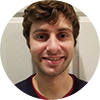##### 16:00 - 16:30 | Efficient classical simulation of random shallow 2D quantum circuits

Authors: John Napp, Rolando La Placa, Alexander Dalzell, Fernando Brandão and Aram Harrow

*Best student paper*

Abstract

Affiliations: Massachusetts Institute of Technology | MIT | California Institute of Technology | California Institute of Technology and Amazon Web Services | Massachusetts Institute of Technology

Extended abstract

Abstract
Random quantum circuits are commonly viewed as hard to simulate classically. In some regimes this has been formally conjectured, and there had been no evidence against the more general possibility that for circuits with uniformly random gates, approximate simulation of typical instances is almost as hard as exact simulation. We prove that this is not the case by exhibiting a shallow circuit family with uniformly random gates that cannot be efficiently classically simulated near-exactly under standard hardness assumptions, but can be simulated approximately for all but a superpolynomially small fraction of circuit instances in time linear in the number of qubits and gates. We furthermore conjecture that sufficiently shallow random circuits are efficiently simulable more generally. To this end, we propose and analyze two simulation algorithms. Implementing one of our algorithms numerically, we give strong evidence that it is efficient both asymptotically and, in some cases, in practice. To argue analytically for efficiency, we reduce the simulation of 2D shallow random circuits to the simulation of a form of 1D dynamics consisting of alternating rounds of random local unitaries and weak measurements -- a type of process that has generally been observed to undergo a phase transition from an efficient-to-simulate regime to an inefficient-to-simulate regime as measurement strength is varied. Using a mapping from quantum circuits to statistical mechanical models, we give evidence that a similar computational phase transition occurs for our algorithms as parameters of the circuit architecture like the local Hilbert space dimension and circuit depth are varied.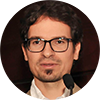##### 16:30 - 17:00 | (Sub)Exponential advantage of adiabatic quantum computation with no sign problem

Authors: Matthew B. Hastings, Umesh Vazirani and András Gilyén

Abstract

Affiliations: Station Q, Microsoft Research, and Microsoft Quantum | Berkeley Quantum Information & Computation Center, UC Berkeley | California Institute of Technology

Extended abstract

Abstract
We demonstrate the possibility of (sub)exponential quantum speedup via a quantum algorithm that follows an adiabatic path of a gapped sparse Hamiltonian with no sign problem. This is in sharp contrast with frustration-free stoquastic Hamiltonians, where no such speedup is possible as shown by Bravyi and Terhal (2008). The Hamiltonian that exhibits this speed-up comes from the adjacency matrix of an undirected graph, and we can view the adiabatic evolution as an efficient $\mathcal{O}(\mathrm{poly}(n))$-time quantum algorithm for finding a specific "EXIT" vertex in the graph given the "ENTRANCE" vertex. On the other hand we show that if the graph is given via an adjacency-list oracle, there is no classical algorithm that finds the "EXIT" with probability greater than $\exp(-n^\delta)$ using at most $\exp(n^\delta)$ queries for $\delta= \frac15 - o(1)$. Our construction of the graph is somewhat similar to the welded-trees'' construction of Childs et al. (2003), but uses additional ideas for simultaneously achieving a spectral gap and a short adiabatic path.

##### 17:00 - 17:30 | Round tables with John Napp and András Gilyén

At the end of the session, you can meet with the speakers directly. Round tables take place in parallel, each speaker having their own meeting.

##### 17:45 - 18:00 | Digital group photo

We would like to commemorate the QIP2021 with the entire community and invite you to join us for a group photo op. This will happen in a Zoom meeting; the link will be shared in the #chit-chat_at_qip Slack channel a few minutes before the event.

##### 18:00 - 18:45 | Mingling

One on one networking session - a great way to meet new people, old friends, and make valuable connections. Participants get randomly matched for one on one talks for 3 minutes. Come over in the MeetAnyway space and try it out.

### Session 4A

Main stage (A)19:00 - 19:30
A Compact Fermion to Qubit Mapping

Authors: Joel Klassen and Charles Derby

Abstract

Affiliations: Phasecraft Ltd. | Phasecraft Ltd. and University College London

Abstract
Mappings between fermions and qubits are valuable constructions in physics. To date only a handful exist. In addition to revealing dualities between fermionic and spin systems, such mappings are indispensable in any simulation of fermionic physics on quantum computers. The number of qubits required per fermionic mode, and the locality of mapped fermionic operators strongly impact the cost of such simulations. Local fermionic encodings are a class of fermion to qubit mappings that map local fermionic operators to local qubit operators. We present a novel local fermionic encoding -- called the compact encoding -- which outperforms all previous local fermionic encodings in both the qubit to mode ratio, and the locality of mapped operators. We demonstrate how this encoding may be applied to any uniform tiling of degree 4 or less, for example square and hexagonal lattices, and to a 3d cubic lattice. In order to characterize the encoding on various lattices we clarify the group theoretic structure underlying the design of such encodings. We also illuminate an elegant relationship between the compact encoding and the toric code, and show how the compact encoding may be understood as condensing the fermionic excitations of the toric code into its code space.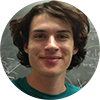Authors: Adrian Chapman and Steven Flammia

Abstract

Affiliations: The University of Sydney | AWS

Extended abstract

Abstract
Exactly solvable models are essential in physics. For many-body spin-1/2 systems, an important class of such models consists of those that can be mapped to free fermions hopping on a graph. We provide a complete characterization of models which can be solved this way. Specifically, we reduce the problem of recognizing such spin models to the graph-theoretic problem of recognizing line graphs, which has been solved optimally. A corollary of our result is a complete set of constant-sized commutation structures that constitute the obstructions to a free-fermion solution. We find that symmetries are tightly constrained in these models. Pauli symmetries correspond to either: (i) cycles on the fermion hopping graph, (ii) the fermion parity operator, or (iii) logically encoded qubits. Clifford symmetries within one of these symmetry sectors, with three exceptions, must be symmetries of the free-fermion model itself. We demonstrate how several exact free-fermion solutions from the literature fit into our formalism and give an explicit example of a new model previously unknown to be solvable by free fermionsAuthors: Adam Bene Watts and J. William Helton

Abstract

Affiliations: MIT | UCSD

Extended abstract

Abstract
We consider 3XOR games with perfect commuting operator strategies. Given any 3XOR game, we show existence of a perfect commuting operator strategy for the game can be decided in polynomial time. Previously this problem was not known to be decidable. Our proof leads to a construction, showing a 3XOR game has a perfect commuting operator strategy iff it has a perfect tensor product strategy using a 3 qubit (8 dimensional) GHZ state. This shows that for perfect 3XOR games the advantage of a quantum strategy over a classical strategy (defined by the quantum-classical bias ratio) is bounded. This is in contrast to the general 3XOR case where the optimal quantum strategies can require high dimensional states and there is no bound on the quantum advantage. To prove these results, we first show equivalence between deciding the value of an XOR game and solving an instance of the subgroup membership problem on a class of right angled Coxeter groups. We then show, in a proof that consumes most of this paper, that the instances of this problem corresponding to 3XOR games can be solved in polynomial time.

20:30 - 21:00
At the end of the session, you can meet with the speakers directly. Round tables take place in parallel, each speaker having their own meeting.

### Session 4B

Stage B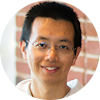19:00 - 19:30
Nearly tight Trotterization of interacting electrons

Authors: Yuan Su, Hsin-Yuan Huang and Earl Campbell

Abstract

Affiliations: QuICS/University of Maryland | Caltech | AWS Center for Quantum Computing

Abstract
We consider simulating quantum systems on digital quantum computers. We show that the performance of quantum simulation can be improved by simultaneously exploiting the commutativity of Hamiltonian, the sparsity of interactions, and the prior knowledge of initial state. We achieve this using Trotterization for a class of correlated electrons that encompasses various physical systems, including the plane-wave-basis electronic structure and the Fermi-Hubbard model. We estimate the simulation error by taking the transition amplitude of nested commutators of Hamiltonian terms within the $\eta$-electron manifold. We develop multiple techniques for bounding the transition amplitude and the expectation of general fermionic operators, which may be of independent interest. We show that it suffices to use $\cO{\frac{n^{5/3}}{\eta^{2/3}}+n^{4/3}\eta^{2/3}}$ gates to simulate electronic structure in the plane-wave basis with $n$ spin orbitals and $\eta$ electrons up to a negligible factor, improving the best previous result in second quantization while outperforming the first-quantized simulation when $\eta=\Om{\sqrt{n}}$. We also obtain an improvement for simulating the Fermi-Hubbard model. We construct concrete examples for which our bounds are almost saturated, giving a nearly tight Trotterization of correlated electrons.19:30 - 20:00
Strongly Universal Hamiltonian Simulators

Authors: Leo Zhou and Dorit Aharonov

Abstract

Affiliations: Harvard University | The Hebrew University of Jerusalem

Abstract
A universal family of Hamiltonians can be used to simulate any local Hamiltonian by encoding its full spectrum as the low-energy subspace of a Hamiltonian from the family. Many spin-lattice model Hamiltonians---such as Heisenberg or XY interaction on the 2D square lattice---are known to be universal. However, the known encodings can be very inefficient, requiring interaction strengths that scales exponentially with system size if the original Hamiltonian have complex, possibly all-to-all connectivity. In this work, we provide an efficient construction by which these universal families are in fact `strongly'' universal; this means that the required interaction strengths as well as all other resources scale polynomially, regardless of the connectivity of the original Hamiltonian. This exponential improvement over previous constructions based on perturbative gadgets is achieved by combining the tools of quantum phase-estimation algorithm and circuit-to-Hamiltonian transformation in a non-perturbative way that only incurs polynomial overhead. Furthermore, we show that 1D Hamiltonians with nearest-neighbor interaction of 8-dimensional particles on a line are also strongly universal Hamiltonian simulators. Our results demonstrate that analog quantum simulation of general Hamiltonians can be made efficient for all target local Hamiltonians; this has potential application for future quantum technologies.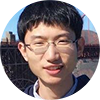20:00 - 20:30
Near-optimal ground state preparation

Authors: Lin Lin and Yu Tong

Abstract

Affiliations: University of California, Berkeley | University of California, Berkeley

Extended abstract

Abstract
Preparing the ground state of a given Hamiltonian and estimating its ground energy are important but computationally hard tasks. However, given some additional information, these problems can be solved efficiently on a quantum computer. We assume that an initial state with non-trivial overlap with the ground state can be efficiently prepared, and the spectral gap between the ground energy and the first excited energy is bounded from below. With these assumptions we design an algorithm that prepares the ground state when an upper bound of the ground energy is known, whose runtime has a logarithmic dependence on the inverse error. When such an upper bound is not known, we propose a hybrid quantum-classical algorithm to estimate the ground energy, where the dependence of the number of queries to the initial state on the desired precision is exponentially improved compared to the current state-of-the-art algorithm proposed in [Ge et al. 2019]. These two algorithms can then be combined to prepare a ground state without knowing an upper bound of the ground energy. We also prove that our algorithms reach the complexity lower bounds by applying it to the unstructured search problem and the quantum approximate counting problem.

20:30 - 21:00
Round tables with Yuan Su, Leo Zhou, and Yu Tong
At the end of the session, you can meet with the speakers directly. Round tables take place in parallel, each speaker having their own meeting.

### Session 4C

Stage C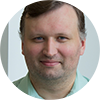Authors: Dmitri Maslov, Jin-Sung Kim, Sergey Bravyi, Theodore J. Yoder and Sarah Sheldon

Abstract

Affiliations: IBM Thomas J. Watson Research Center | IBM Almaden Research Center | IBM Thomas J. Watson Research Center | IBM Thomas J. Watson Research Center | IBM Almaden Research Center

Extended abstract

Abstract
Quantum computations promise the ability to solve problems intractable in the classical setting. Restricting the types of computations considered often allows to establish a provable theoretical advantage by quantum computations, and later demonstrate it experimentally. In this paper, we consider space-restricted computations, where input is a read-only memory and only one (qu)bit can be computed on. We show that n-bit symmetric Boolean functions can be implemented exactly through the use of quantum signal processing as restricted space quantum computations using O(n^2) gates, but some of them may only be evaluated with probability 1/2+O(n/sqrt{2}^n) by analogously defined classical computations. We experimentally demonstrate computations of 3-, 4-, 5-, and 6-bit symmetric Boolean functions by quantum circuits, leveraging custom two-qubit gates, with algorithmic success probability exceeding the best possible classically. This establishes and experimentally verifies a different kind of quantum advantage---one where quantum scrap space is more valuable than analogous classical space---and calls for an in-depth exploration of space-time tradeoffs in quantum circuits.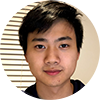Authors: Nathan Ju, Daniel Grier and Luke Schaeffer

Abstract

Affiliations: University of Illinois, Urbana-Champaign | University of Waterloo | University of Waterloo

Extended abstract

Abstract
Recent work by Bravyi et al. constructs a relation problem that a noisy constant-depth quantum circuit (QNC^0) can solve with near certainty (probability 1 - o(1)), but that any bounded fan-in constant-depth classical circuit (NC^0) fails with some constant probability. We show that this robustness to noise can be achieved in the other low-depth quantum/classical circuit separations in this area. In particular, we show a general strategy for adding noise tolerance to the interactive protocols of Grier and Schaeffer. As a consequence, we obtain an unconditional separation between noisy QNC^0 circuits and AC^0[p] circuits for all primes p \geq 2, and a conditional separation between noisy QNC^0 circuits and log-space classical machines under a plausible complexity-theoretic conjecture. A key component of this reduction is showing average-case hardness for the classical simulation tasks---that is, showing that a classical simulation of the quantum interactive task is still powerful even if it is allowed to err on some constant fraction of inputs. We show that is possible even for quantum tasks which are parity-L-hard to simulate. To do this, we borrow techniques from randomized encodings used in cryptography.20:00 - 20:30
Fast simulation of planar Clifford circuits

Authors: David Gosset, Daniel Grier, Alex Kerzner and Luke Schaeffer

A general quantum circuit can be simulated in exponential time on a classical computer. If it has a planar layout, then a tensor-network contraction algorithm due to Markov and Shi has a runtime exponential in the square root of its size, or more generally exponential in the treewidth of the underlying graph. Separately, Gottesman and Knill showed that if all gates are restricted to be Clifford, then there is a polynomial time simulation. We combine these two ideas and show that treewidth and planarity can be exploited to improve Clifford circuit simulation. Our main result is a classical algorithm with runtime scaling asymptotically as ~$n^{\omega/2}<n^{1.19}$ which samples from the output distribution obtained by measuring all $n$ qubits of a planar graph state in given Pauli bases. Here $\omega$ is the matrix multiplication exponent. We also provide a classical algorithm with the same asymptotic runtime which samples from the output distribution of any constant-depth Clifford circuit in a planar geometry. Our work improves known classical algorithms with cubic runtime. A key ingredient is a mapping which, given a tree decomposition of some graph $G$, produces a Clifford circuit with a structure that mirrors the tree decomposition and which emulates measurement of the quantum graph state corresponding to $G$. We provide a classical simulation of this circuit with the runtime stated above for planar graphs and otherwise $n t^{\omega-1}$ where $t$ is the width of the tree decomposition. Our algorithm incorporates two subroutines which may be of independent interest. The first is a matrix-multiplication-time version of the Gottesman-Knill simulation of multi-qubit measurement on stabilizer states. The second is a new classical algorithm for solving symmetric linear systems over $\mathbb{F}_2$ in a planar geometry.